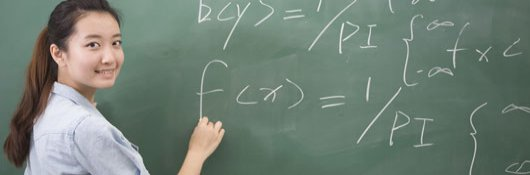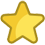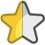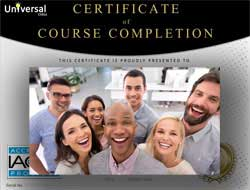# Online Class: Precalculus 101

This course provides a bridge between algebra and calculus, guiding students through the underlying mathematical concepts needed to understand and appreciate calculus.Self-Paced, Online Class
Library Subscription

• 19
Lessons
• 21
Exams &
Assignments
• 35
Hours
average time
• 3.5
CEUs
•
Video Audit
Available

## Course Description

Calculus is a mathematical tool that is required in a variety of scientific, technical, and other professional fields. Because it is a higher-level area of math, many people think it is a subject that they can never understand. Unfortunately, such an attitude limits an individual's career choices, particularly in a technology-focused society.

This course provides a bridge between algebra and calculus, guiding students through the underlying mathematical concepts needed to understand and appreciate calculus. The course reviews real and complex numbers, functions, and equations and inequalities. It then goes into some detail on specific types of functions, such as polynomials, exponentials, and logarithms. It also provides a derivation of the principles of trigonometry on the basis of simple right-triangle and circle geometry. After reviewing vectors, polar coordinates, and conic sections, the material sets up calculus proper by examining limits as well as sequences and series.

The final lessons of the course use the basic principles of algebra to derive the two key tools of calculus: differentiation (the derivative) and integration (the integral). At the end of this course, students will be prepared to study calculus in greater depth, further investigating and applying derivatives and integrals to solve a variety of math, physics, and other problems.

## Course Lessons

Average Lesson Rating:• #### Lesson 1 - Real and Complex Numbers

In this lesson, we begin the process of setting a foundation for understanding calculus by looking briefly at real and complex numbers. These are the sets of values that we will be studying at length in this course.
• #### Lesson 2 - Functions I

Having introduced the real and complex number sets in the previous lesson, we now begin to look in depth at series of operations on numbers, or more particularly, numbers and variables.
• #### Lesson 3 - Functions II

Having defined functions in the previous lesson, we now look in more detail at the characteristics of functions. These concepts are critical and will underlie the remainder of this course.
• #### Lesson 4 - Equations and Inequalities

Having looked in detail at functions, the course now turns to equations and inequalities: two mathematical expressions that relate different algebraic relations involving numbers, variables, or both.
• #### Lesson 5 - Polynomials and Rational Functions I

Having looked in general at characteristics of functions and their relationship to equations and inequalities, we now turn to a common class of functions, polynomials, and their close cousins, rational functions.x`
• #### Lesson 6 - Polynomials and Rational Functions II

In the previous lesson, we defined and discussed some characteristics of polynomials and rational functions. In this lesson, we will look at these functions in slightly more depth.
• #### Lesson 7 - Exponential and Logarithmic Functions

This lesson turns away from polynomials (and their close cousins, rational functions) and looks at another type of function: exponential and logarithmic functions. We consider the basic rules of exponents and logarithms and apply them to simple functions.
• #### Lesson 8 - Trigonometric Functions I

We now consider another class of functions called trigonometric ("trig") functions. These functions are useful in a variety of contexts involving triangles and circles. This lesson considers the origin of these functions in right triangles and looks at so
• #### Lesson 9 - Trigonometric Functions II

In the previous lesson, we reviewed the origin of trigonometric functions in the context of right triangles. Interestingly, however, trig functions also have applicability to circles. The present lesson will focus on circles and the relationship of circle
• #### Lesson 10 - Trigonometric Functions III

In this lesson, we will look at a few more aspects of trigonometry, including trigonometric identities and inverse trigonometric functions.
• #### Lesson 11 - Vectors I

In this lesson, we introduce a new quantity: the vector. Vectors-unlike simple numbers (scalars) that have only a magnitude-have both a magnitude (length) and direction. We will explore how to represent vector quantities, as well as how to add and subtr
• #### Lesson 12 - Vectors II

In the previous lesson, we introduced vectors as quantities with a magnitude and direction. In this lesson, we look more deeply into vectors to flesh out some of the concepts introduced previously.
• #### Lesson 13 - Polar Coordinates

In this lesson, we introduce a new type of coordinate system: polar coordinates. Polar coordinates are one example of the many alternative coordinate systems that can be devised; these alternatives can greatly simplify certain problems.
• #### Lesson 14 - Conic Sections I

In this lesson, we introduce conic sections and show how they are related to cross sections of a cone (hence their name). The basic characteristics-algebraic and graphical-of two conic sections are discussed in detail: circles and parabolas. The next less
• #### Lesson 15 - Conic Sections II

This lesson continues our examination of conic sections, focusing specifically on ellipses and hyperbolas. The end of the lesson presents a practical way of relating all the conics we have studied thus far by way of the motion of celestial objects.
• #### Lesson 16 - Limits

In this lesson, we introduce limits, a topic fundamental to understanding differentiation and integration-two critical aspects of calculus. Limits are generally intuitive, but a solid ability to use them is important to the remainder of the course.
• #### Lesson 17 - Sequences and Series

The current lesson switches our focus slightly to look briefly at sequences and series. These mathematical constructs play an important role in calculus and other branches of mathematics.
• #### Lesson 18 - Introduction to Derivatives

Having covered all the necessary mathematical background, the final two lessons of the course turn to two fundamental concepts in calculus: the derivative and the integral. Although we will not go into great depth on these subjects, we will show how they
• #### Lesson 19 - Introduction to Integrals

In the previous lesson, we covered one of two major aspects of calculus: the derivative. For this closing lesson to the course, we will look briefly at the other of these two concepts: the integral.

## Learning Outcomes

By successfully completing this course, students will be able to:
• Define, identify and demonstrate use of real and complex numbers.
• Define, identify and demonstrate use of functions.
• Define, identify and demonstrate use of equations and inequalities.
• Define, identify and demonstrate use of polynomials and rational functions.
• Define, identify and demonstrate use of exponential and logarithmic functions.
• Define, identify and demonstrate use of trigonometric functions.
• Define, identify and demonstrate use of vectors.
• Define, identify and demonstrate use of polar coordinates.
• Define, identify and demonstrate use of conic sections.
• Define, identify and demonstrate use of limits, sequences, and series.
• Define, identify and demonstrate use of derivatives and integrals.
• Demonstrate mastery of lesson content at levels of 70% or higher.• Document Your Lifelong Learning Achievements
• Earn an Official Certificate Documenting Course Hours and CEUs
• Verify Your Certificate with a Unique Serial Number OnlineCourse Title: Precalculus 101
Course Number: 9770456
Lessons Rating: 4.5 / 5 Stars (1,068 votes)
Languages: English - United States, Canada and other English speaking countries
Category:
Availability: This course is online and available in all 50 states including: California, Florida, Georgia, Illinois, New York, Pennsylvania, Ohio, and Texas.
Last Updated: February 2022
Course Type: Self-Paced, Online Class
CEU Value: 3.5 IACET CEUs (Continuing Education Units)
CE Accreditation: Universal Class, Inc. has been accredited as an Authorized Provider by the International Association for Continuing Education and Training (IACET).